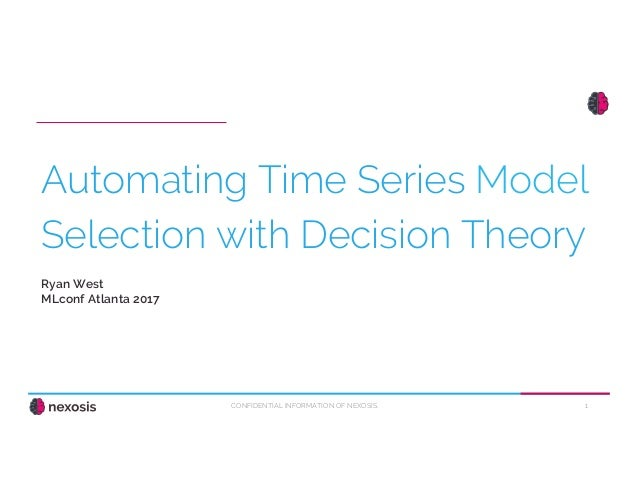Successfully reported this slideshow.Upcoming SlideShare
×

# Ryan West, Machine Learning Engineer, Nexosis at MLconf ATL 2017

342 views

Published on

Codifying Data Science Intuition: Using Decision Theory to Automate Time Series Model Selection:
While models generated from cross-sectional data can utilize cross-validation for model selection, most time series models cannot be cross-validated due to the temporal structure of the data used to create them. It is possible to employ a rolling cross-validation technique, however this process is computationally expensive and provides no indication of the long-term forecast accuracies of the models.

The purpose of this talk is to elaborate how decision theory can be used to automate time series model selection in order to streamline the manual process of validation and testing. By creating consecutive, temporally independent holdout sets, performance metrics for each model’s prediction on each holdout set are fed into a decision function to select an unbiased model. The decision function helps minimize the poorest performance of each model across all holdout sets in order to counteract the possibility of choosing a model that overfits or underfits the holdout sets. Not only does this process improve forecast accuracy, but it also reduces computation time by only requiring the creation of a fixed number of proposed forecasting models.

Published in: Technology
• Full Name
Comment goes here.

Are you sure you want to Yes No• Be the first to like this

### Ryan West, Machine Learning Engineer, Nexosis at MLconf ATL 2017

1. 1. 1CONFIDENTIAL INFORMATION OF NEXOSIS. Automating Time Series Model Selection with Decision Theory Ryan West MLconf Atlanta 2017
2. 2. 2CONFIDENTIAL INFORMATION OF NEXOSIS. K-Folds Cross-Validation Rolling Time Series Cross-Validation https://en.wikipedia.org/wiki/Cross-validation_(statistics) https://robjhyndman.com/hyndsight/tscv/
3. 3. 3CONFIDENTIAL INFORMATION OF NEXOSIS. Problem Visualized Total Test Set Model 1 Forecast Model 2 Forecast Model M Forecast Test Set Subset 1 Test Set Subset N Test Set Subset 1 Test Set Subset N Test Set Subset 1 Test Set Subset N ....... ....... .............. Minimize: Maximize:
4. 4. 4CONFIDENTIAL INFORMATION OF NEXOSIS. Formulation o minimax(x1, x2, …, xM) o xi = a variable of N possible values o error metric calculated with N test sets and forecast of model i min s subject to: s ≥ x1 s ≥ x2 … s ≥ xM Equivalent to:
5. 5. 5CONFIDENTIAL INFORMATION OF NEXOSIS. Alternative Problem Total Test Set Test Set Subset 1 Test Set Subset 2 Test Set Subset N Model 1 Forecast Model M Forecast Model 1 Forecast Model M Forecast Model 1 Forecast Model M Forecast ....... ..................... Maximize: Minimize:
6. 6. 6CONFIDENTIAL INFORMATION OF NEXOSIS. Alternative Formulation o maximin(x1, x2, …, xN) o xi = a variable of M possible values o error metric calculated with the forecasts of M models and test set i Equivalent to: max s subject to: s ≤ x1 s ≤ x2 … s ≤ xN
7. 7. 7CONFIDENTIAL INFORMATION OF NEXOSIS. Experiment o 856 time series of daily retail sales data o 7 exogenous variables per time series o e.g. promotions, holidays, indicator variables of store open or closed o 38 possible models o Testing forecast accuracy of different model selection techniques
8. 8. 8CONFIDENTIAL INFORMATION OF NEXOSIS. Model Selection Techniques o Selection using ensembling o Single test set for model selection o Additional holdout set o Selection based on maximin of error metric o Multiple test sets for model selection o Additional holdout set o Selection based on minimizing error metric o Single test set for model selection o Additional holdout set
9. 9. 9CONFIDENTIAL INFORMATION OF NEXOSIS. Partial Autocorrelation o Strongly seasonal time series
10. 10. 10CONFIDENTIAL INFORMATION OF NEXOSIS. Error Metric Visualization (MAE)
11. 11. 11CONFIDENTIAL INFORMATION OF NEXOSIS. Error Metric Visualization (RMSE)
12. 12. 12CONFIDENTIAL INFORMATION OF NEXOSIS. Error Metric Visualization (RMSPE)
13. 13. 13CONFIDENTIAL INFORMATION OF NEXOSIS. Error Metric Visualization (sMAPE)
14. 14. 14CONFIDENTIAL INFORMATION OF NEXOSIS. Forecast Accuracy Average RMSPE* on Holdout Set Model Selection Technique Feature Engineering 0.382 Minimizing RMSPE on test set No 0.223 Naïve median weekly seasonal predictions No 0.215 Maximin of RMSPE on test set subsets Yes 0.204 Minimizing RMSPE on test set Yes 0.191 Ensemble Averaging Yes *RMSPE = Root Mean Squared Percentage Error
15. 15. 15CONFIDENTIAL INFORMATION OF NEXOSIS. Thank You!